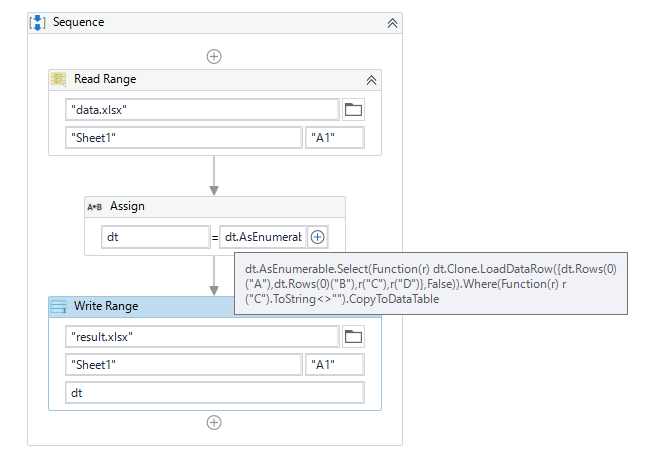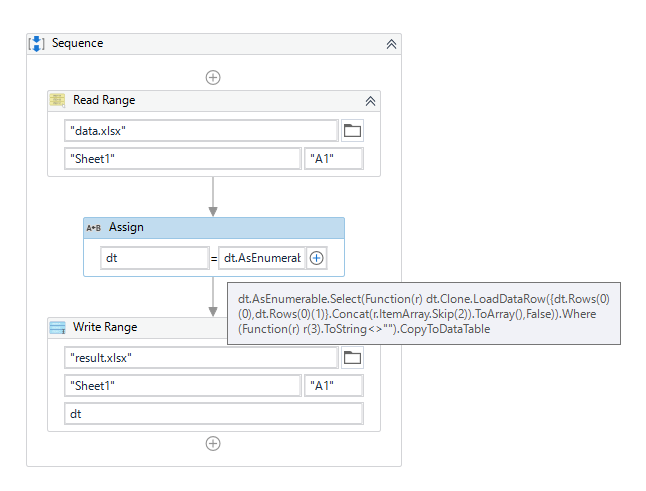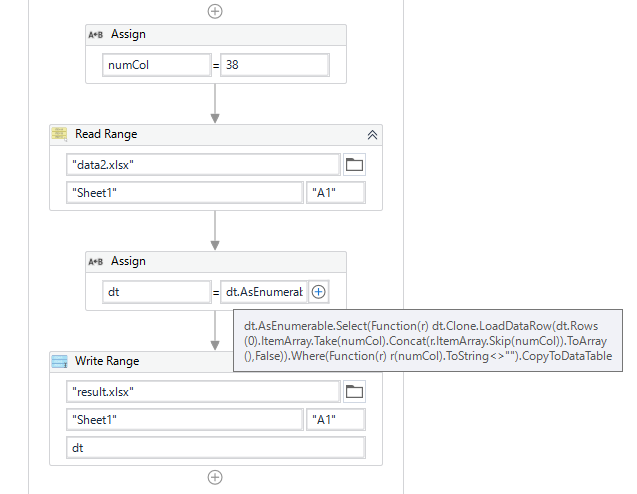# How to organize data tables

hello
I have a question for you.
In the image below, I want to copy the data table on the left as the number of rows in A and B columns, as on the right. And in the case of C and D, there are parts where the lines do not match, so I want to match them.
How can I make the data table on the left look like the one on the right?

Thank you!!

Hi,

Can you try the following sample?``````dt = dt.AsEnumerable.Select(Function(r) dt.Clone.LoadDataRow({dt.Rows(0)("A"),dt.Rows(0)("B"),r("C"),r("D")},False)).Where(Function(r) r("C").ToString<>"").CopyToDataTable
``````

Sample20221129-6.zip (8.6 KB)

Regards,

Columns are simply expressed in the figure, but the number of columns is very large in actual data.
Is there any way other than adding the header directly?Hi,

If the first 2 columns always is same group, the following will work, even if number of remaining column is vary.`````` dt = dt.AsEnumerable.Select(Function(r) dt.Clone.LoadDataRow({dt.Rows(0)(0),dt.Rows(0)(1)}.Concat(r.ItemArray.Skip(2)).ToArray(),False)).Where(Function(r) r(2).ToString<>"").CopyToDataTable
``````

Sample20221129-6v2.zip (8.7 KB)

If the above doesn’t fit for you, can you share some examples?

Regards,

Thank you for your quick response.
I just tested it and it doesn’t respond.
The number of my columns is 41 in total, and there are 38 columns like A and B as shown in the picture.
Only 3 columns appear like C and D.

Hi,

All right. Can you try the following?

``````dt = dt.AsEnumerable.Select(Function(r) dt.Clone.LoadDataRow(dt.Rows(0).ItemArray.Take(numCol).Concat(r.ItemArray.Skip(numCol)).ToArray(),False)).Where(Function(r) r(numCol).ToString<>"").CopyToDataTable
``````

Sample20221129-6v3.zip (8.7 KB)

Regards,

thank you I entered the following, but the error [the source contains no datarow] occurs.

Hi,

Is your input sheet like the following, right?

If we set 38 as numCol in the previous sample,Sample20221129-6v4.zip (15.7 KB)

the result will be the following.

Is this same as what you expect?

If so, can you check if your data is same as the above?

Regards,

oh!! Thank you very much.
I misunderstood the column number. (An error occurred because the middle header was empty :))

It’s been resolved.
thank you!

1 Like

This topic was automatically closed 3 days after the last reply. New replies are no longer allowed.